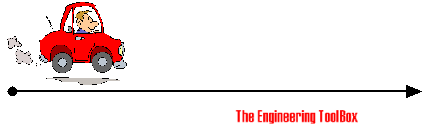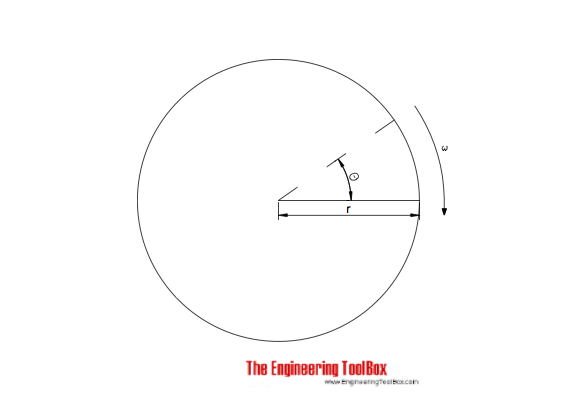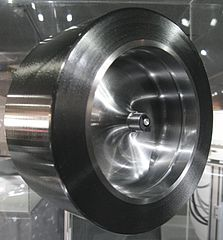Engineering ToolBox - Resources, Tools and Basic Information for Engineering and Design of Technical Applications!

# Formulas of Motion - Linear and Circular

## Linear and angular (rotation) acceleration, velocity, speed and distance.

### Linear Motion FormulasAverage velocity/speed of a moving object can be calculated as

v = s / t                            (1a)

where

v = velocity or speed (m/s, ft/s)

s = linear distance traveled (m, ft)

t = time (s)

• distance is the length of the path a body follows in moving from one point to another - displacement is the straight line distance between the initial and final positions of the body
• we use velocity and speed interchangeable - but be aware that speed is a measure of how fast or slow a distance is covered, the rate at which distance is covered - velocity is a vector, specifying how fast or slow a distance is covered and the direction

If acceleration is constant then velocity can be expressed as:

v = v0 + a t                            (1b)

where

v0 = initial linear velocity (m/s, ft/s)

a = acceleration (m/s2, ft/s2)

Linear distance can be expressed as (if acceleration is constant):

s = v0 t + 1/2 a t2                                       (1c)

Combining 1b and 1c to express the final velocity

v = (v02 + 2 a s)1/2                                    (1d)

Velocity can be expressed as (velocity is variable)

v = ds / dt (1f)

where

ds = change in distance (m, ft)

dt = change in time (s)

Acceleration can be expressed as

a = dv / dt                                   (1g)

where

dv = change in velocity (m/s, ft/s)

#### Example - a Marathon Run

If a marathon - 42195 m - is run in amazing 2:03:23 (7403 seconds) (Wilson Kipsang, Kenya - September 29, 2013 Berlin Marathon) - the average speed can be calculated

v = (42195 m) / (7403 s)

= 5.7 m/s

= 20.5 km/h

#### Example - Acceleration of a Car

A car accelerates from 0 km/h to 100 km/h in 10 seconds. The acceleration can be calculated by transforming (1b) to

a = (v - v0) / t

= ( (100 km/h) (1000 m/km) / (3600 s/h) - (0 km/h) (1000 m/km) / (3600 s/h) ) / (10 s)

= 2.78 (m/s2)

### Linear Motion Calculators

#### Average velocity

s - distance (m, km, ft, miles)

t - time used (s, h)

#### Distance

v0 - initial velocity (m/s, ft/s)

a - acceleration (m/s2, ft/s2)

t - time used (s, h)

#### Final Velocity

v0 - initial velocity (m/s, ft/s)

a - acceleration (m/s2, ft/s2)

s - distance (m, ft)

#### Acceleration

v - final velocity (m/s, ft/s)

v0 - initial velocity (m/s, ft/s)

t - time used (s)

### Circular Motion - Rotation#### Angular Velocity

Angular velocity can be expressed as (angular velocity = constant):

ω = θ / t                           (2)

where

t = time (s)

Angular velocity and rpm:

ω = 2 π n / 60                        (2a)

where

n = revolutions per minute (rpm)

π = 3.14...

The tangential velocity of a point in angular velocity - in metric or imperial units like m/s or ft/s - can be calculated as

v = ω r                              (2b)

where

v = tangential velocity (m/s, ft/s, in/s)

r = distance from center to the point (m, ft, in)

#### Example - Tangential Velocity of a Bicycle Tire

A 26 inches bicycle wheel rotates with an angular velocity of π radians/s (0.5 turn per second). The tangential velocity of the tire can be calculated as

v = (π radians/s) ((26 inches) / 2)

= 40.8 inches/s

#### Angular Velocity and Acceleration

Angular velocity can also be expressed as (angular acceleration = constant):

ω = ωo + α t                               (2c)

where

ωo = angular velocity at time zero (rad/s)

α = angular acceleration or deceleration (rad/s2)

#### Angular Displacement

Angular distance can be expressed as (angular acceleration is constant):

θ = ωo t + 1/2 α t2                                     (2d)

Combining 2a and 2c:

ω = (ωo2 + 2 α θ)1/2

#### Angular Acceleration

Angular acceleration can be expressed as:

α = dω / dt = d2θ / dt2                                    (2e)

where

dθ = change of angular distance (rad)

dt = change in time (s)

##### Example - Flywheel DecelerationBy Geni (Photo by User:geni) [GFDL or CC-BY-SA-3.0-2.5-2.0-1.0], via Wikimedia Commons

A flywheel is slowed down from 2000 rpm (revolutions/min) to 1800 rpm in 10 s. The deceleration of the flywheel can be calculated as

α = ((2000 rev/min)  - (1800 rev/min)) (0.01667 min/s) (2 π rad/rev) / (10 s)

= 120 degrees/s2

#### Angular Moment - or Torque

Angular moment or torque can be expressed as:

T = α I                                 (2f)

where

T = angular moment or torque (N m)

I = Moment of inertia (lbm ft2, kg m2)

## Related Topics

• Dynamics - Motion - velocity and acceleration, forces and torque.
• Mechanics - Forces, acceleration, displacement, vectors, motion, momentum, energy of objects and more.

## Engineering ToolBox - SketchUp Extension - Online 3D modeling!

Add standard and customized parametric components - like flange beams, lumbers, piping, stairs and more - to your Sketchup model with the Engineering ToolBox - SketchUp Extension - enabled for use with the amazing, fun and free SketchUp Make and SketchUp Pro .Add the Engineering ToolBox extension to your SketchUp from the SketchUp Pro Sketchup Extension Warehouse!

Translate

## Privacy

We don't collect information from our users. Only emails and answers are saved in our archive. Cookies are only used in the browser to improve user experience.

Some of our calculators and applications let you save application data to your local computer. These applications will - due to browser restrictions - send data between your browser and our server. We don't save this data.

## Citation

• Engineering ToolBox, (2005). Formulas of Motion - Linear and Circular. [online] Available at: https://www.engineeringtoolbox.com/motion-formulas-d_941.html [Accessed Day Mo. Year].

Modify access date.

. .

#### Scientific Online Calculator3 10